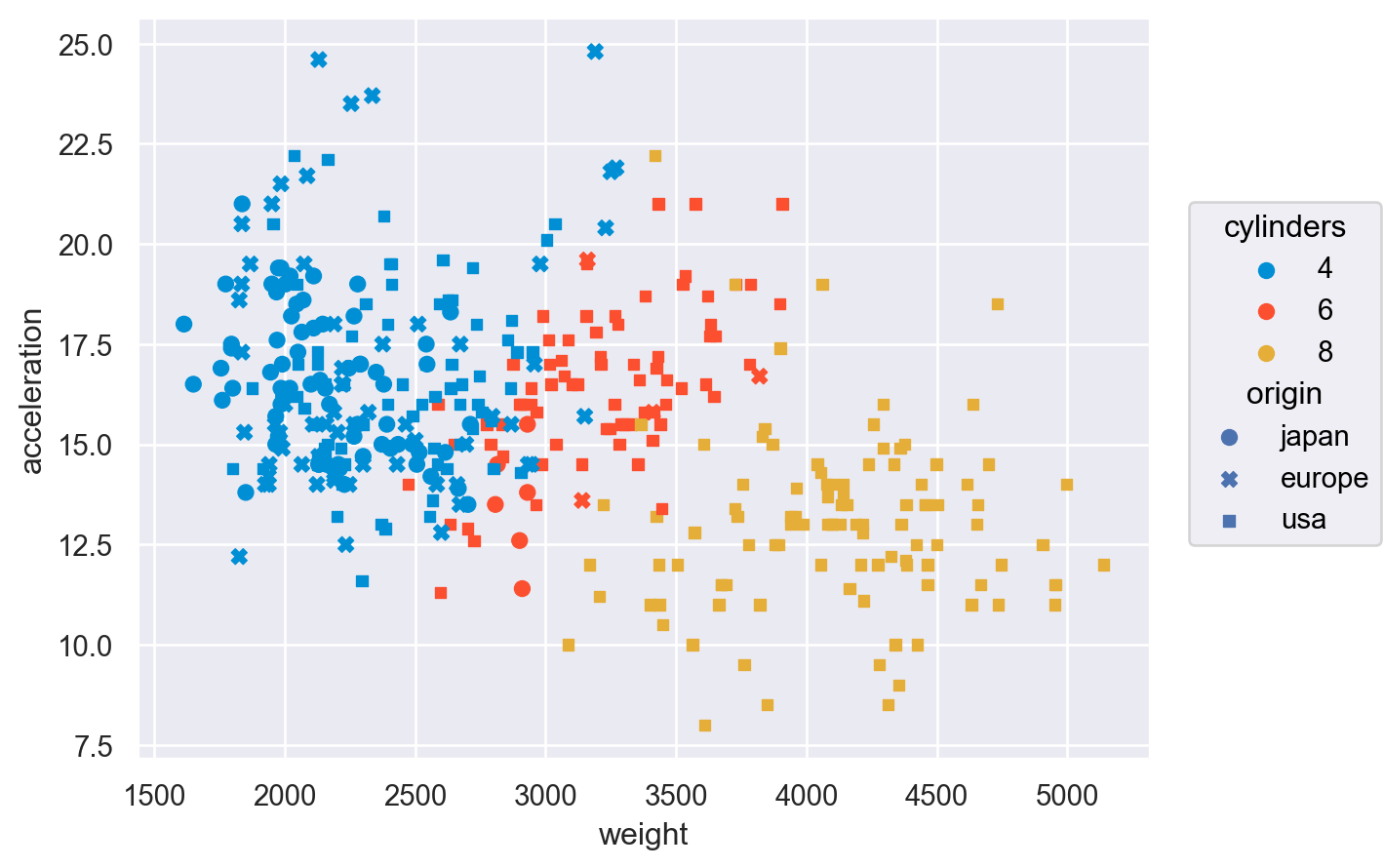# seaborn.objects.Plot.scale#

Plot.scale(**scales)#

Specify mappings from data units to visual properties.

Keywords correspond to variables defined in the plot, including coordinate variables (`x`, `y`) and semantic variables (`color`, `pointsize`, etc.).

A number of “magic” arguments are accepted, including:
• The name of a transform (e.g., `"log"`, `"sqrt"`)

• The name of a palette (e.g., `"viridis"`, `"muted"`)

• A tuple of values, defining the output range (e.g. `(1, 5)`)

• A dict, implying a `Nominal` scale (e.g. `{"a": .2, "b": .5}`)

• A list of values, implying a `Nominal` scale (e.g. `["b", "r"]`)

For more explicit control, pass a scale spec object such as `Continuous` or `Nominal`. Or pass `None` to use an “identity” scale, which treats data values as literally encoding visual properties.

Examples

Passing the name of a function, such as `"log"` or `"symlog"` will set the scale’s transform:

```p1 = so.Plot(diamonds, x="carat", y="price")
```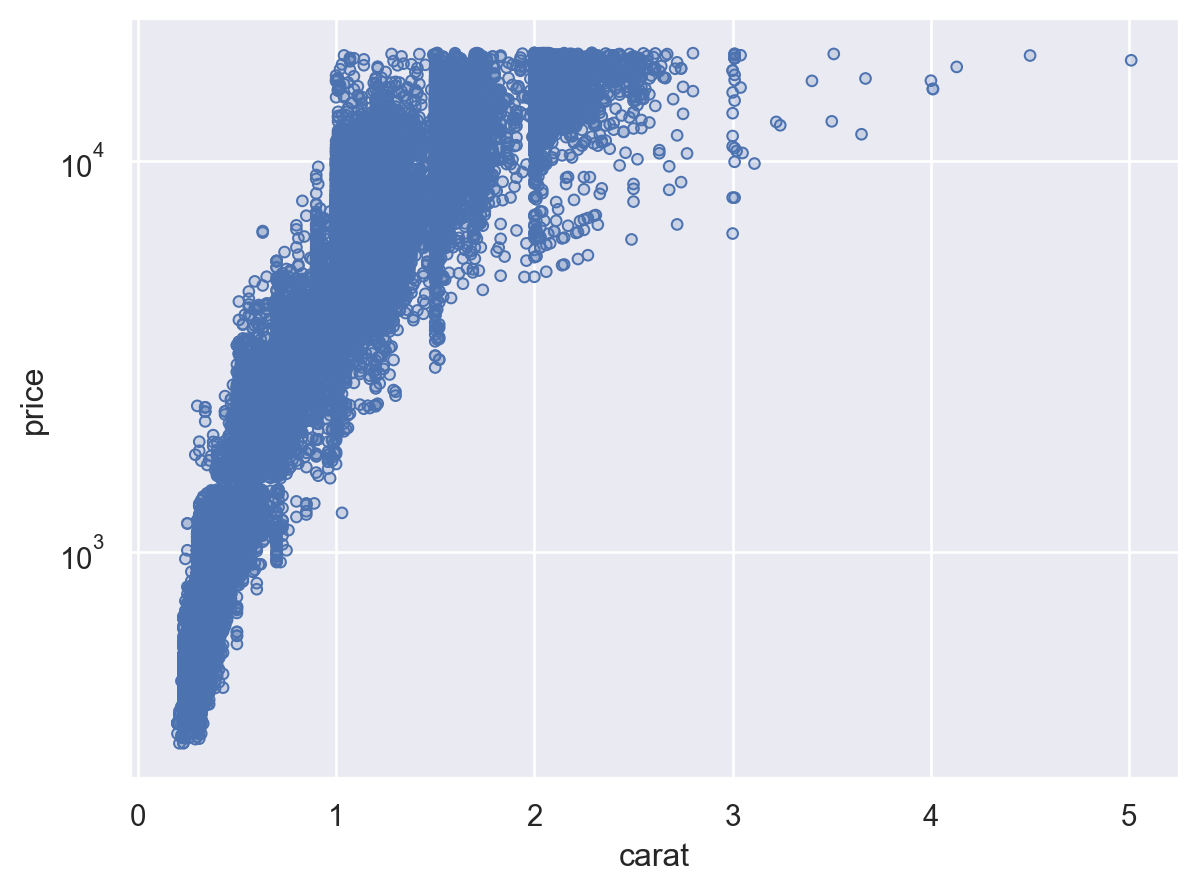String arguments can also specify the the name of a palette that defines the output values (or “range”) of the scale:

```p1.add(so.Dots(), color="clarity").scale(color="crest")
```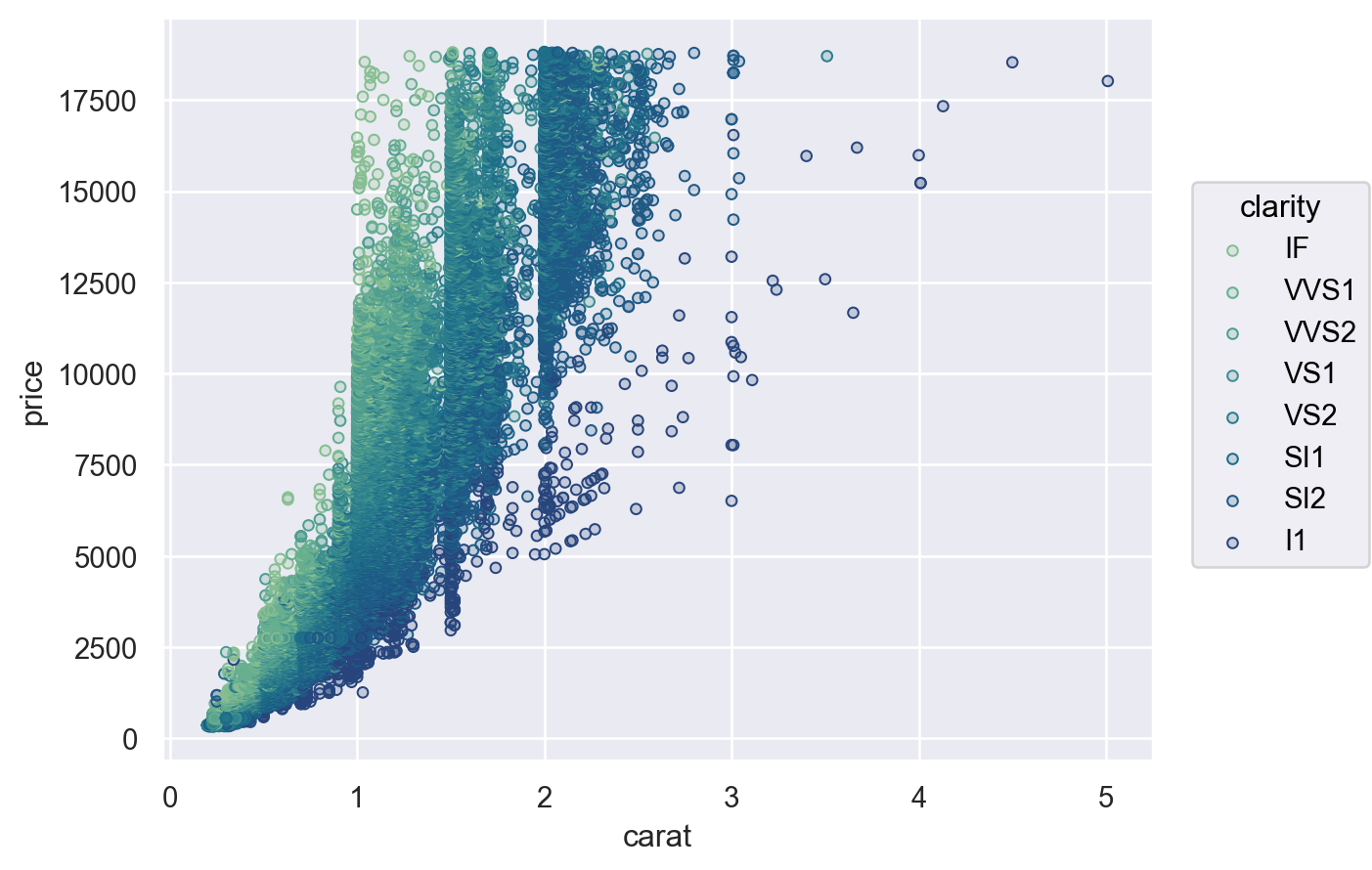The scale’s range can alternatively be specified as a tuple of min/max values:

```p1.add(so.Dots(), pointsize="carat").scale(pointsize=(2, 10))
```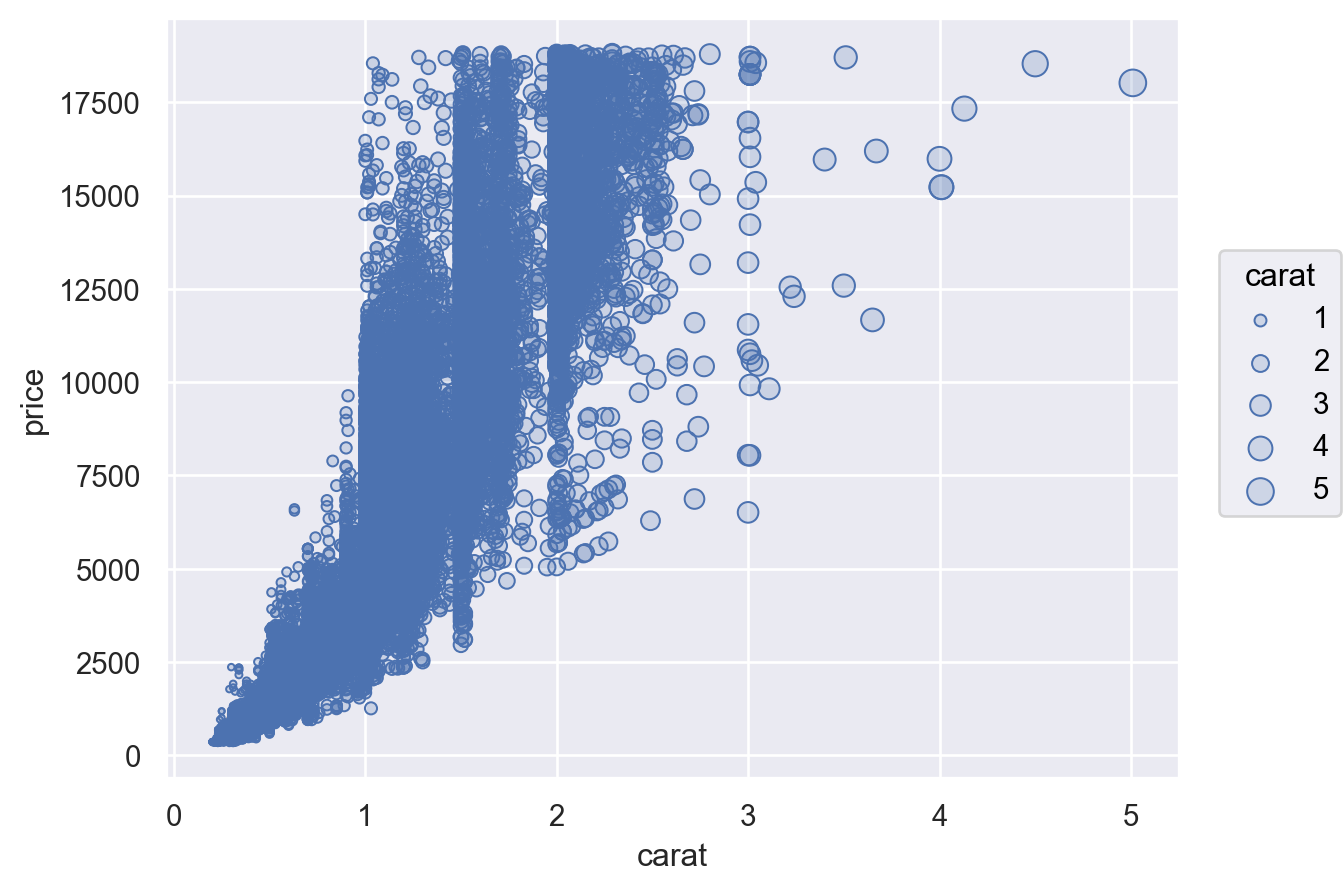The tuple format can also be used for a color scale:

```p1.add(so.Dots(), color="carat").scale(color=(".4", "#68d"))
```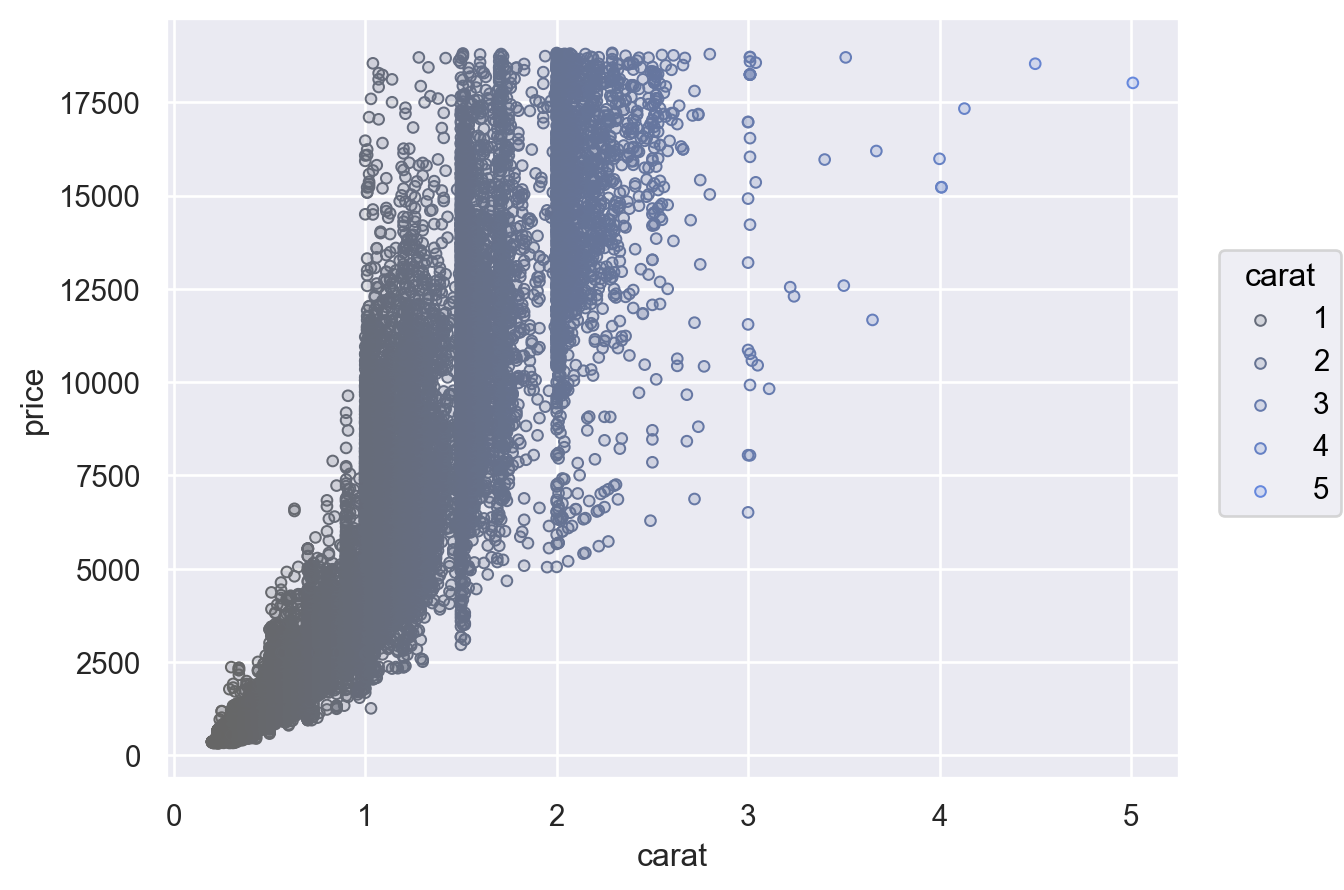For more control pass a scale object, such as `Continuous`, which allows you to specify the input domain (`norm`), output range (`values`), and nonlinear transform (`trans`):

```(
.scale(color=so.Continuous((".4", "#68d"), norm=(1, 3), trans="sqrt"))
)
```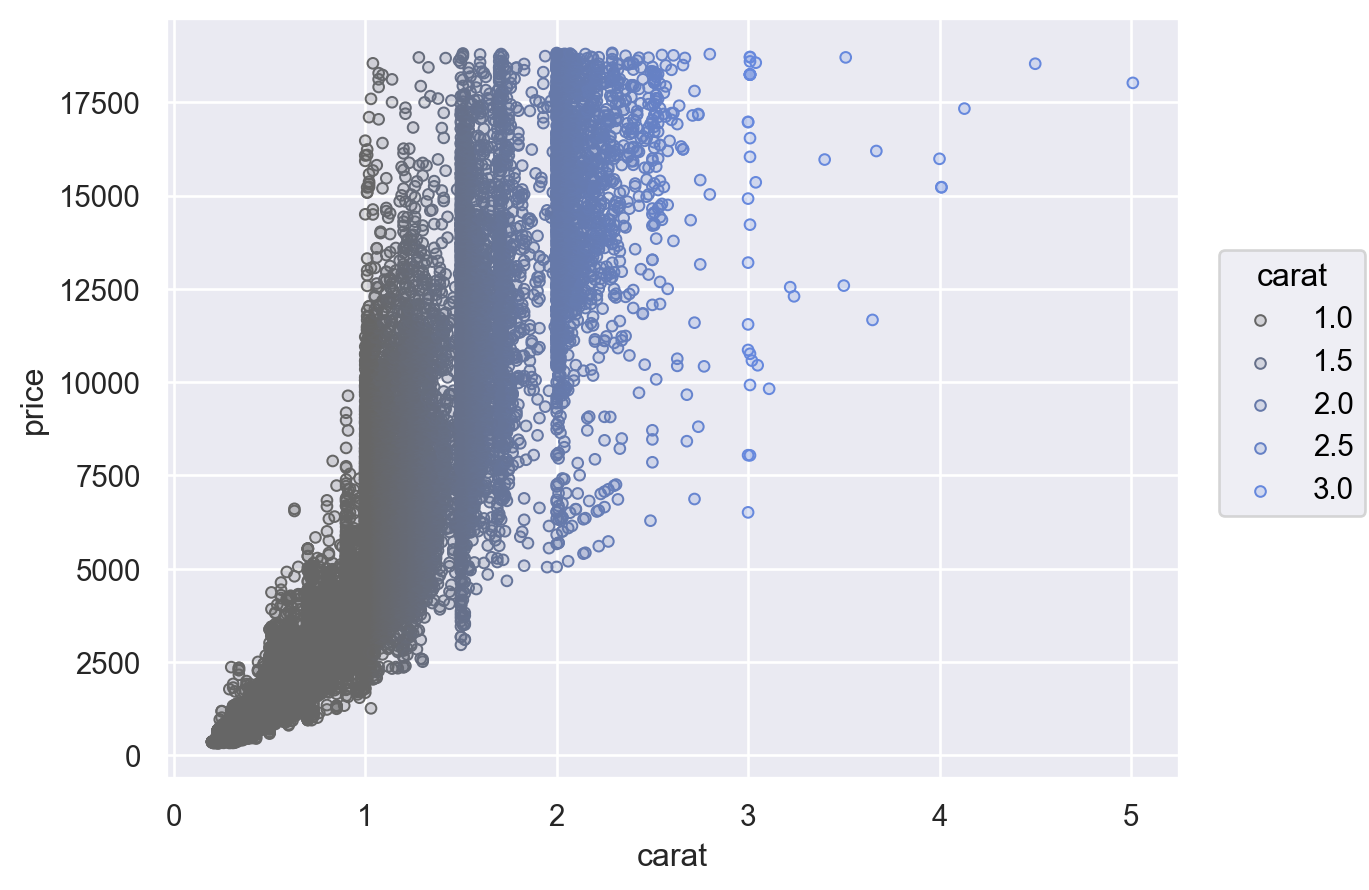The scale objects also offer an interface for configuring the location of the scale ticks (including in the legend) and the formatting of the tick labels:

```(
.scale(
x=so.Continuous(trans="sqrt").tick(every=.5),
y=so.Continuous().label(like="\${x:g}"),
color=so.Continuous("ch:.2").tick(upto=4).label(unit=""),
)
.label(y="")
)
```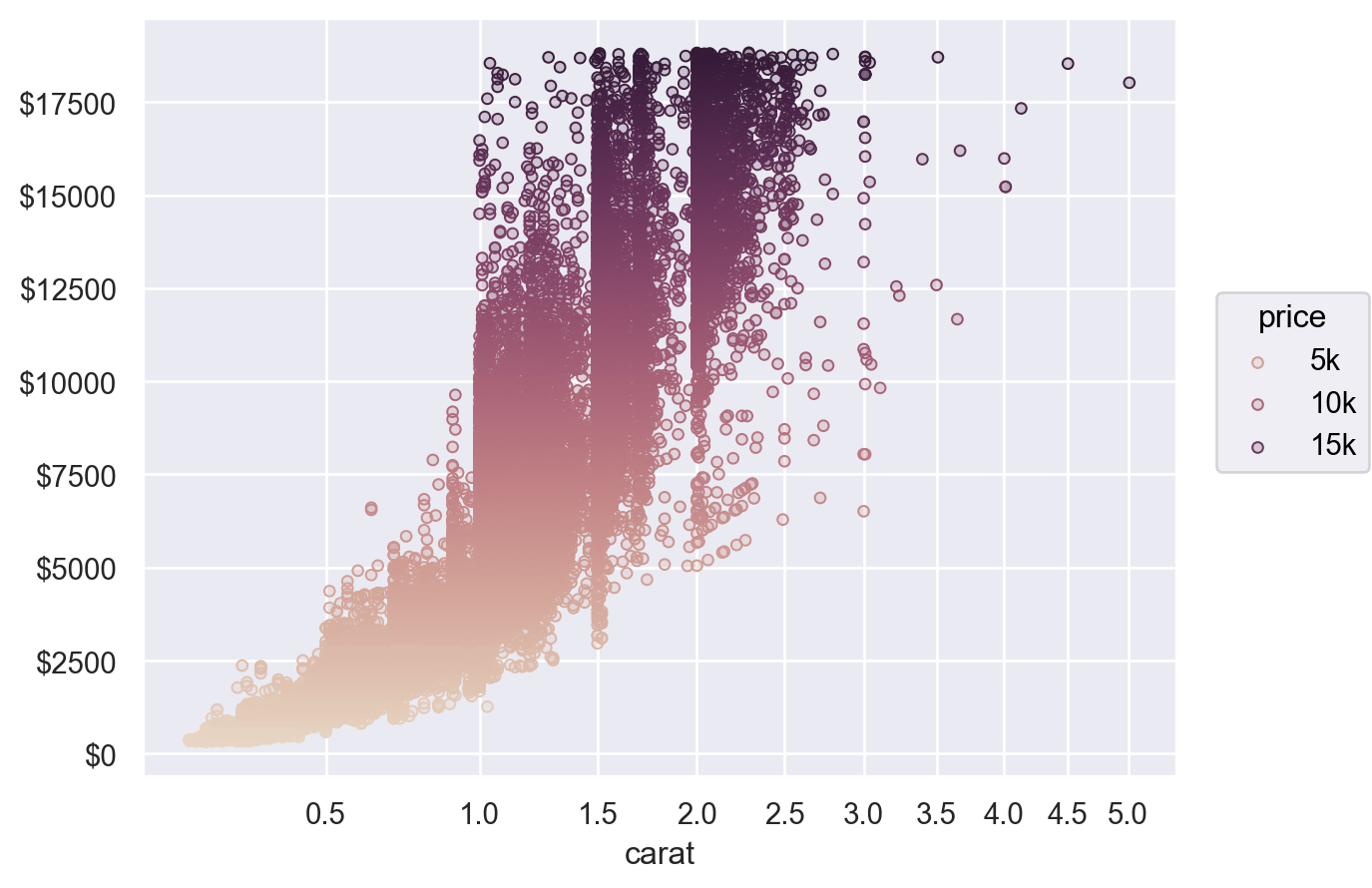If the scale includes a nonlinear transform, it will be applied before any statistical transforms:

```(
.scale(y="log")
.limit(y=(250, 25000))
)
```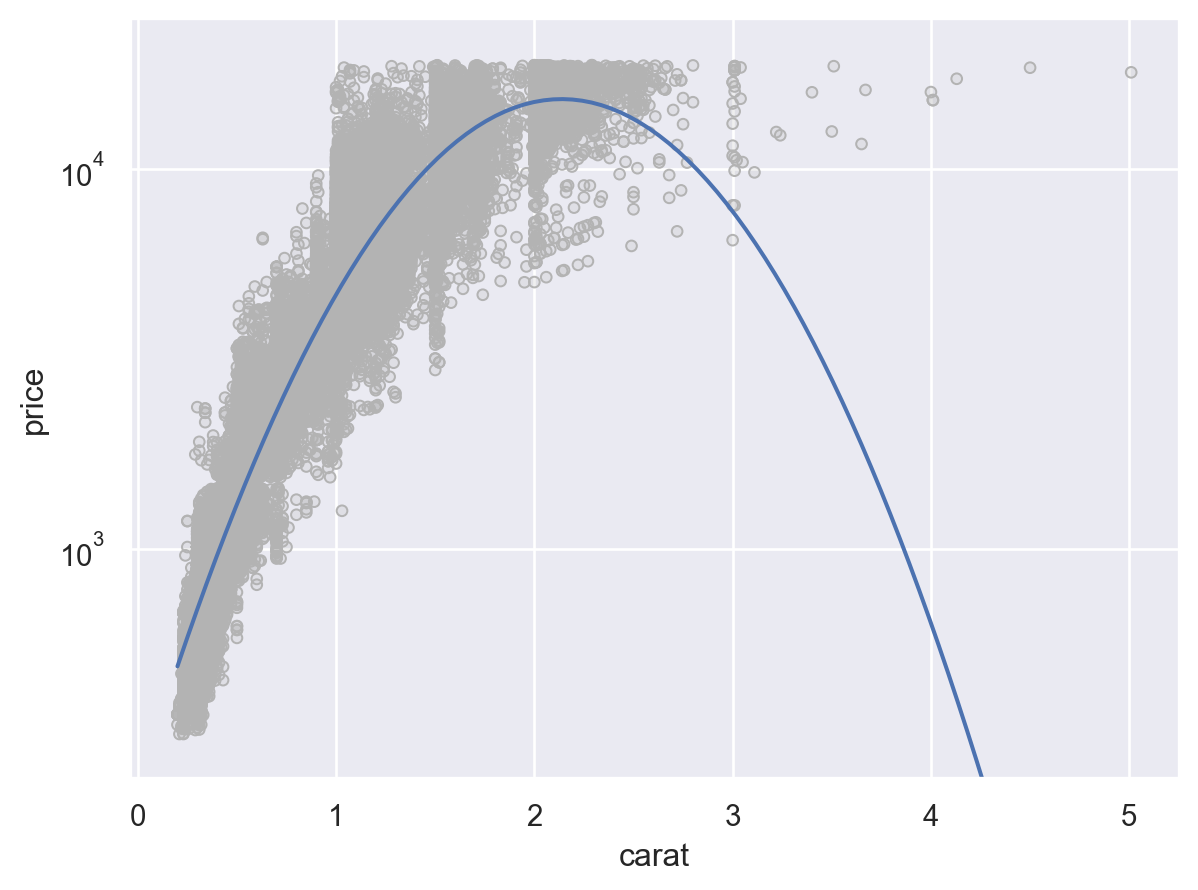The scale is also relevant for when numerical data should be treated as categories. Consider the following histogram:

```p2 = so.Plot(mpg, "cylinders").add(so.Bar(), so.Hist())
p2
```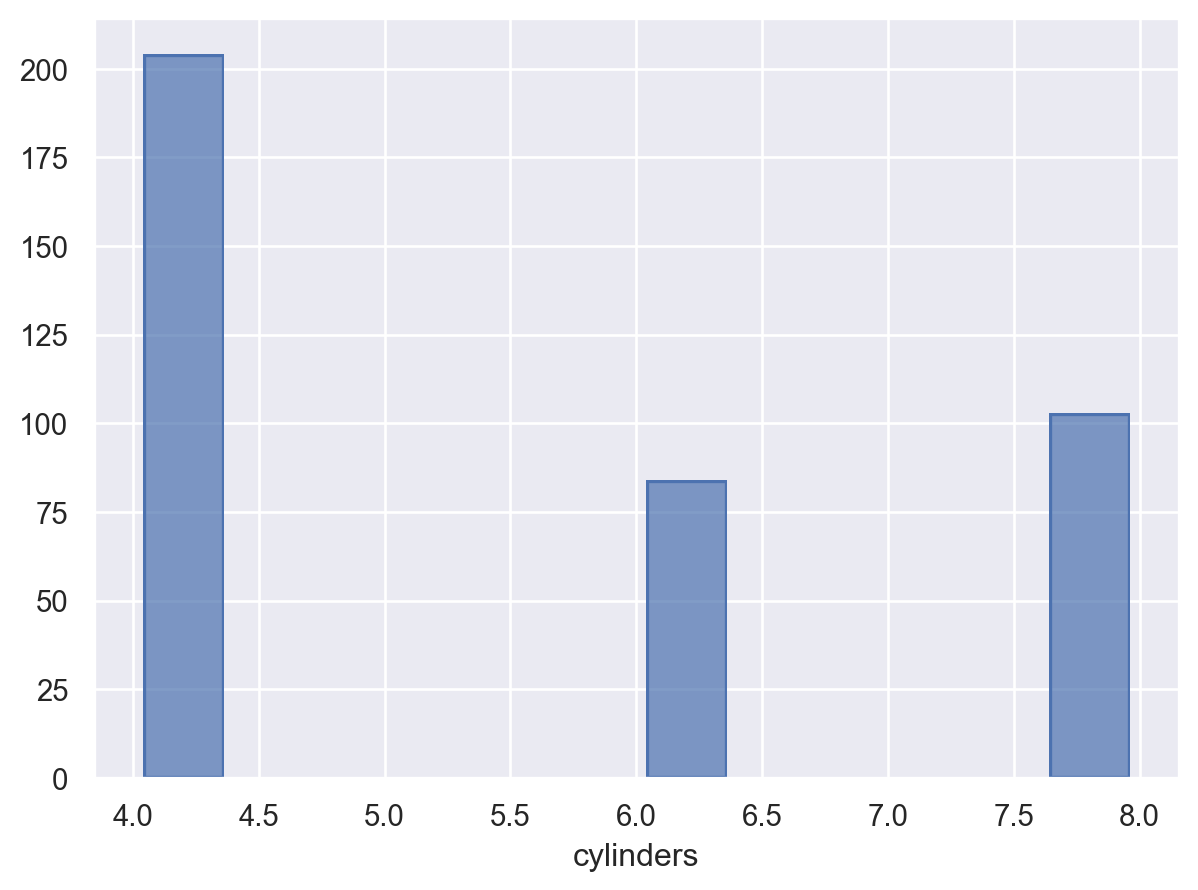By default, the plot gives `cylinders` a continuous scale, since it is a vector of floats. But assigning a `Nominal` scale causes the histogram to bin observations properly:

```p2.scale(x=so.Nominal())
```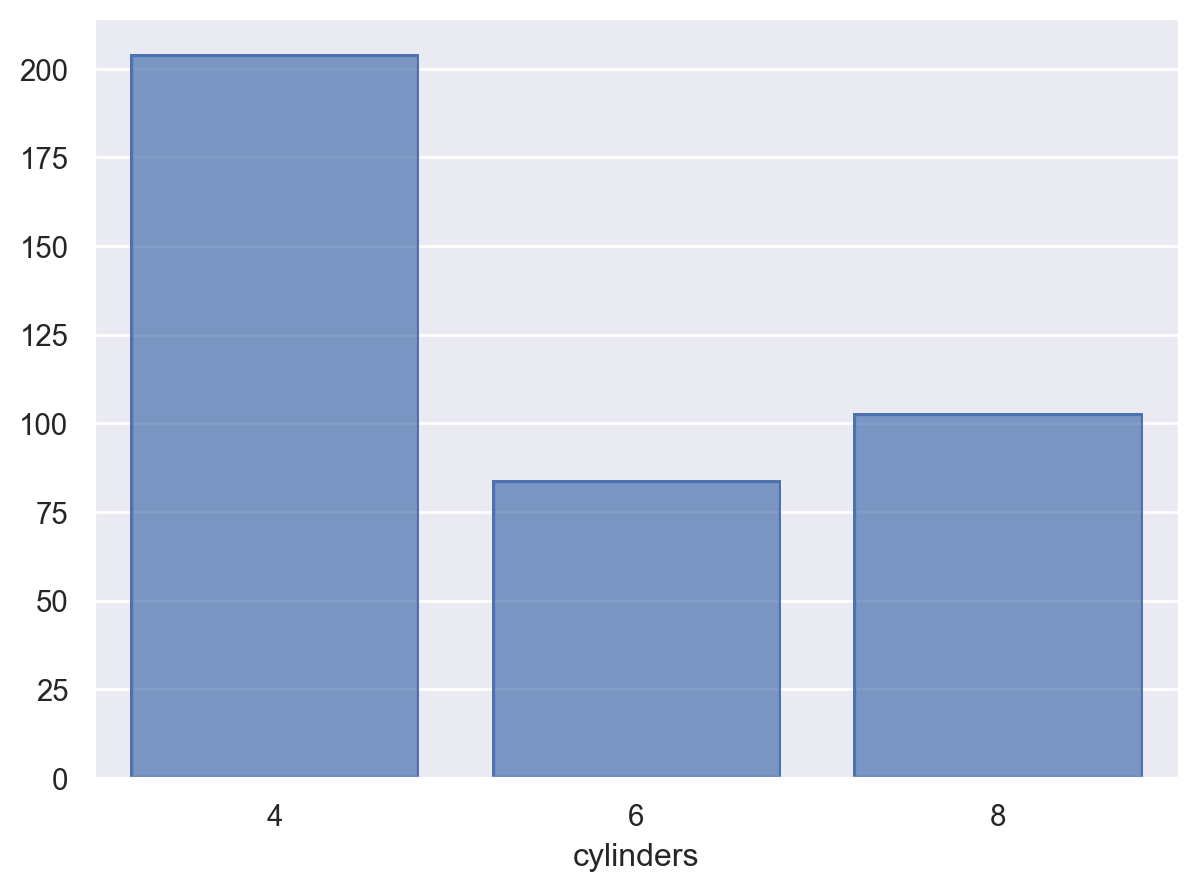The default behavior for semantic mappings also depends on input data types and can be modified by the scale. Consider the sequential mapping applied to the colors in this plot:

```p3 = (
so.Plot(mpg, "weight", "acceleration", color="cylinders")
)
p3
```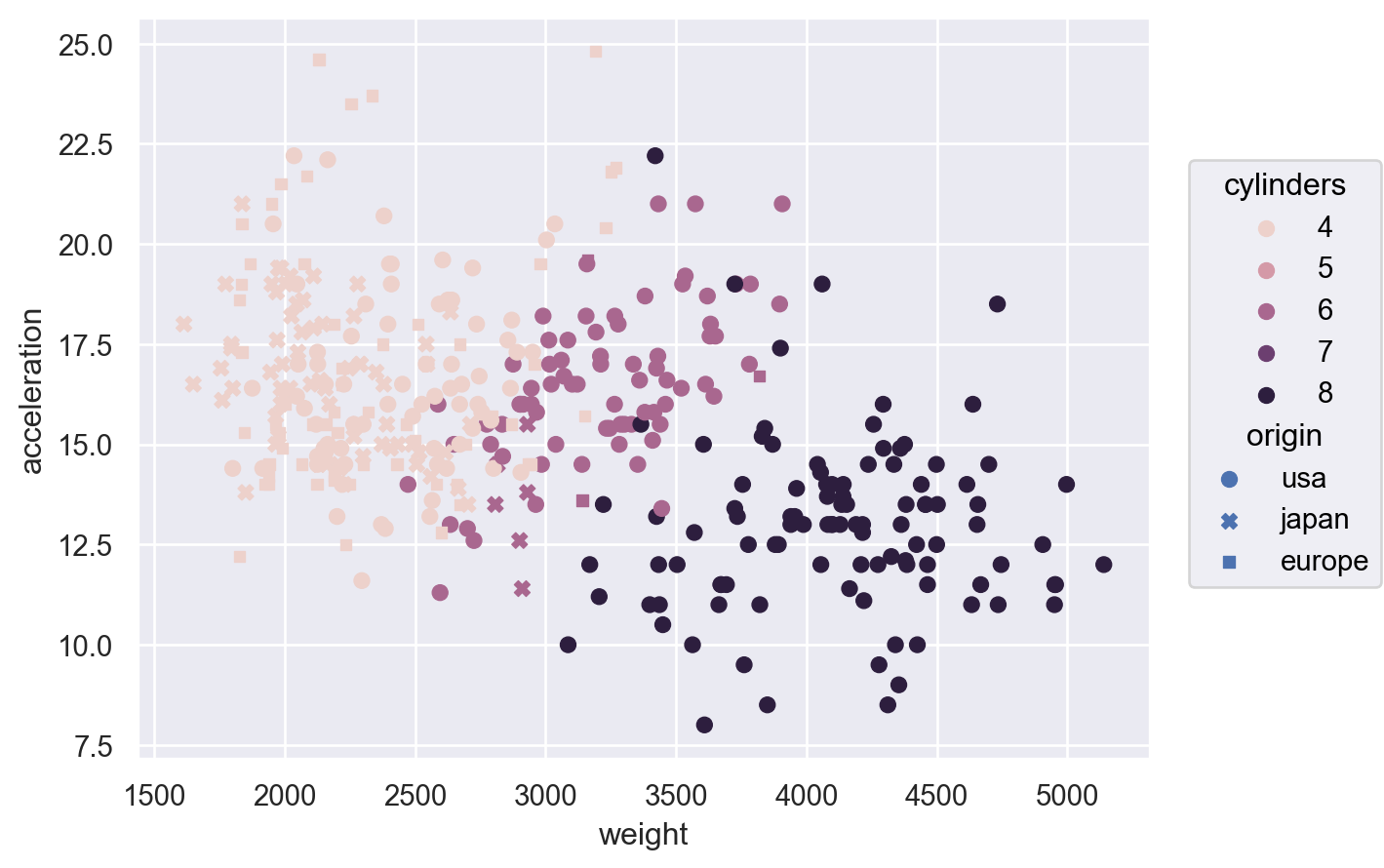Passing the name of a qualitative palette will select a `Nominal` scale:

```p3.scale(color="deep")
```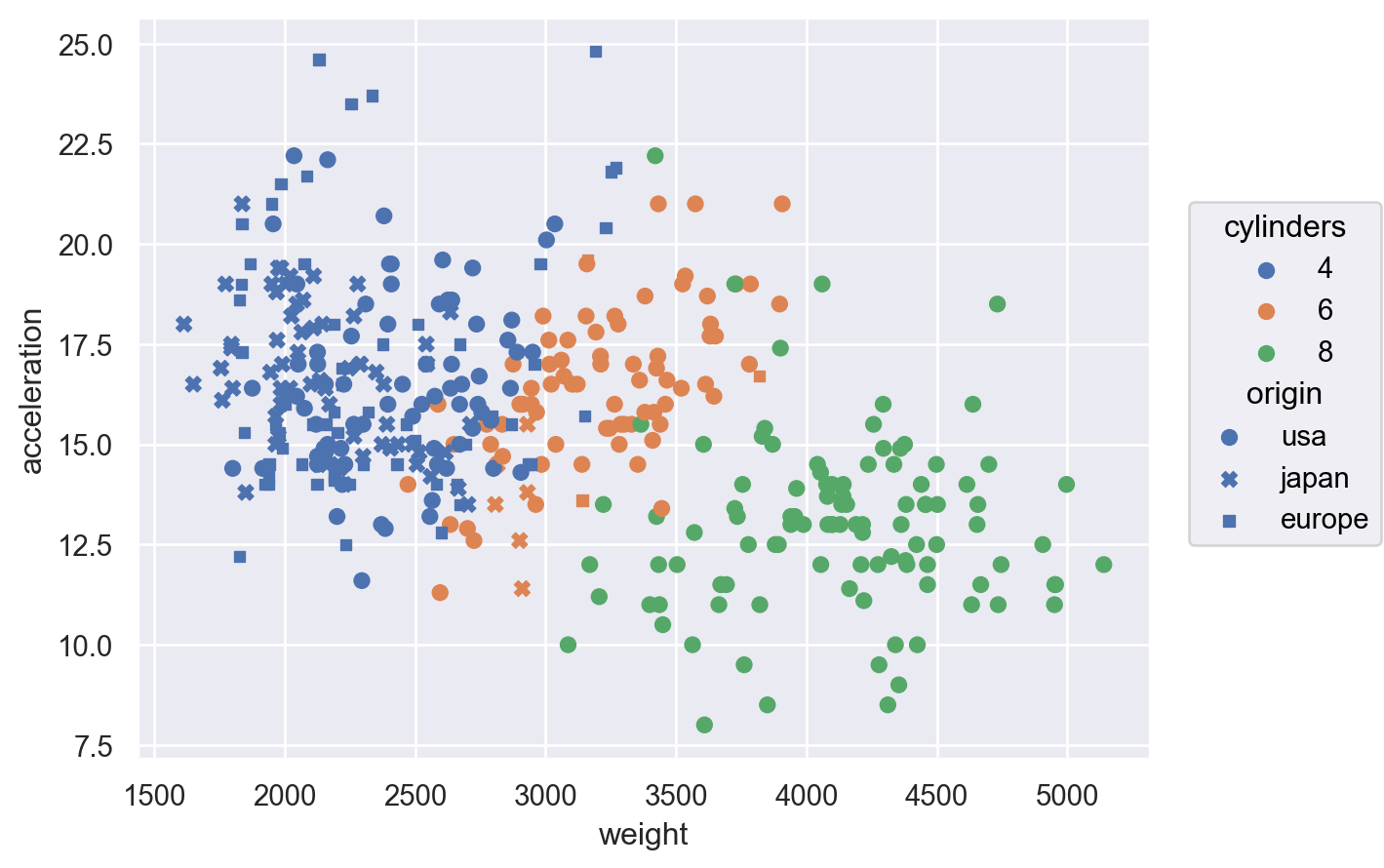A `Nominal` scale is also implied when the output values are given as a list or dictionary:

```p3.scale(
color=["#49b", "#a6a", "#5b8"],
marker={"japan": ".", "europe": "+", "usa": "*"},
)
```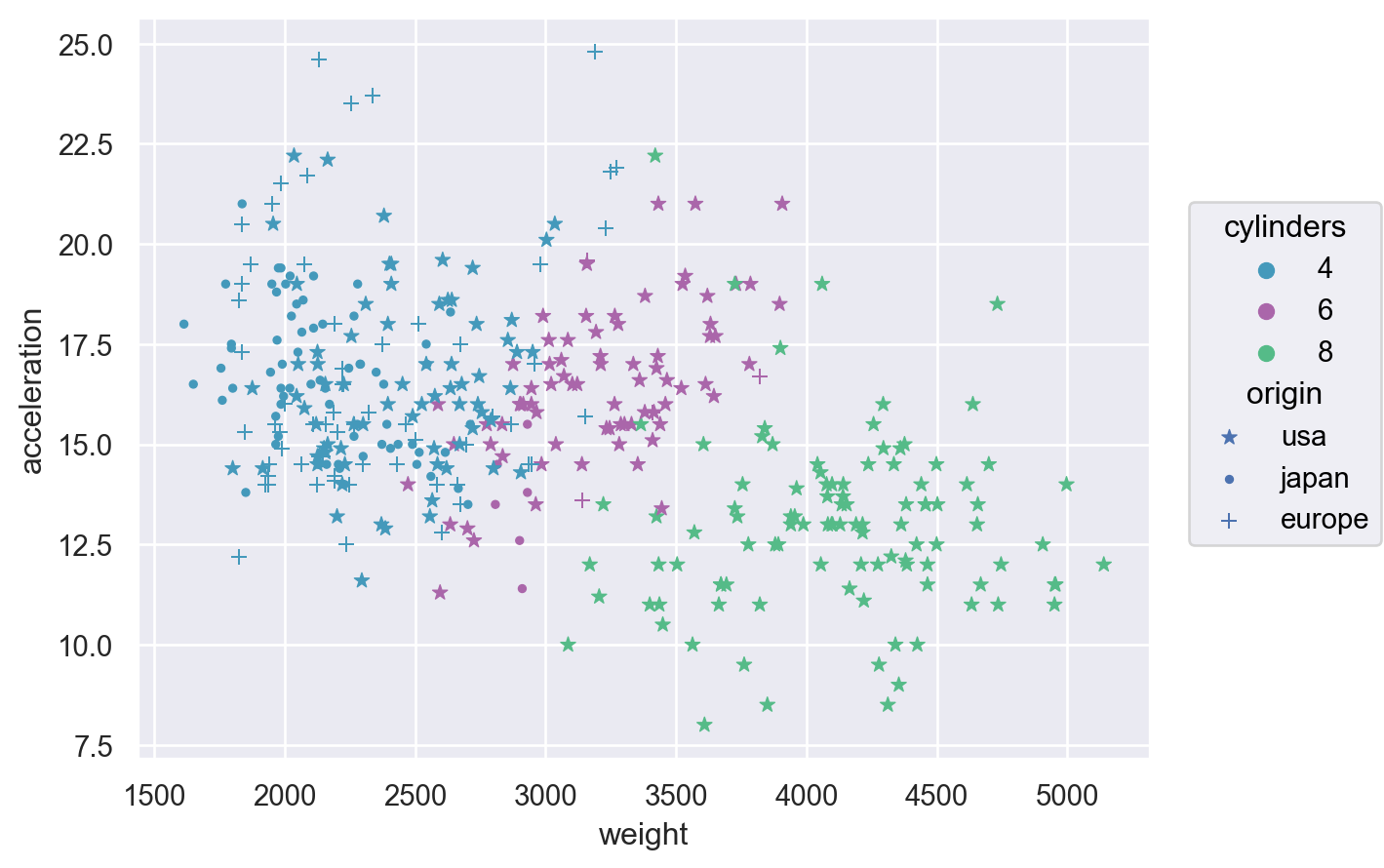Pass a `Nominal` object directly to control the order of the category mappings:

```p3.scale(
color=so.Nominal(["#008fd5", "#fc4f30", "#e5ae38"]),
marker=so.Nominal(order=["japan", "europe", "usa"])
)
```